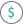#DOLLARDE (PQL - xl)

This function converts a fraction dollar price to a decimal dollar price

• Library: PQL \ Spreadsheet \ Financial
• Compatibility: Any content (regardless of data source) in the Tabulate spreadsheet module

#### Syntax

DOLLARDE(fractional dollar, fraction)

##### Function Arguments
 Name Description Type Optional fractional dollar Numeric value expressed as a fraction Number fraction Denominator of the fraction; if not an integer, the number is truncated Number